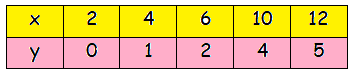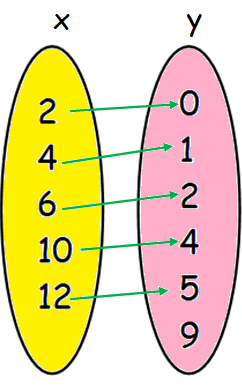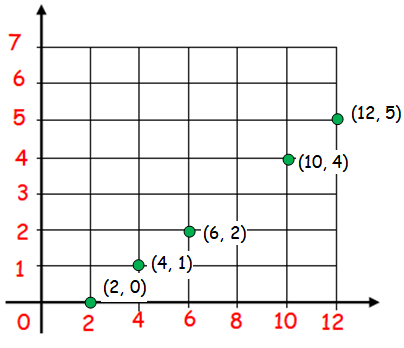## Pages

### Let f : A -> B be a function defined by f (x) = (x/2) - 1 where A = {2, 4, 6, 10, 12}, B = {0, 1, 2, 4, 5, 9} .

Let f : A -> B be a function defined by f (x) =  (x/2) - 1 where A = {2, 4, 6, 10, 12}, B = {0, 1, 2, 4, 5, 9} .
Represent f by
(i) set of ordered pairs; (ii) a table; (iii) an arrow diagram; (iv) a graph
Solution :
(i) set of ordered pairs
f (x) =  (x/2) - 1
Set of ordered pairs  = {(2, 0) (4, 1) (6, 2) (10, 4) (12, 5)}
(ii) a table(iii) an arrow diagram;(iv) a graph